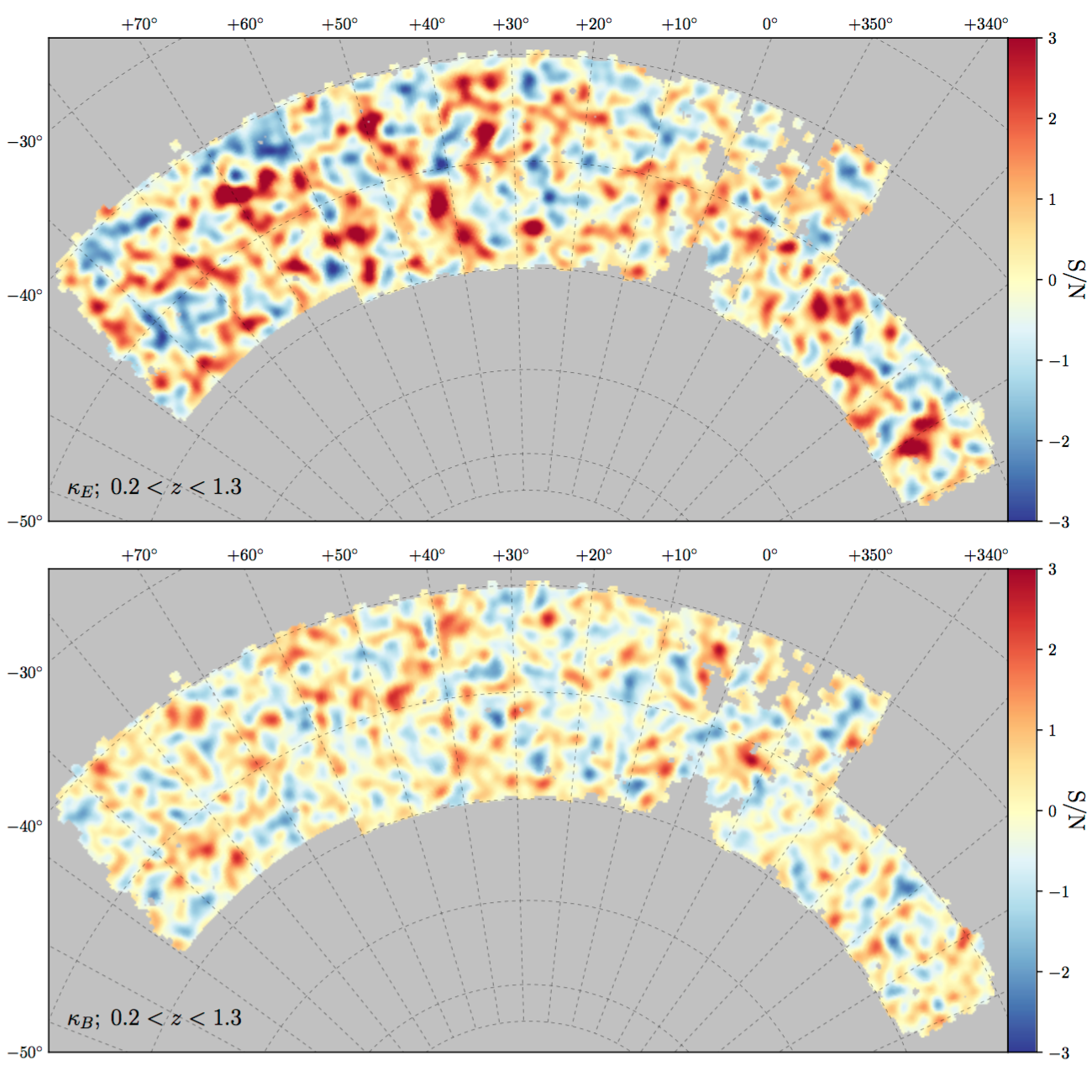# Dark Energy Survey Year 1 Results: Curved-Sky Weak Lensing Mass MapWe construct the largest curved-sky galaxy weak lensing mass map to date from the DES first-year (DES Y1) data. The map, about 10 times larger than previous work, is constructed over a contiguous $\approx1,500$deg$^2$, covering a comoving volume of $\approx10$Gpc$^3$. The effects of masking, sampling, and noise are tested using simulations. We generate weak lensing maps from two DES Y1 shear catalogs, Metacalibration and Im3shape, with sources at redshift $0.2<z<1.3,$ and in each of four bins in this range. In the highest signal-to-noise map, the ratio between the mean signal-to-noise in the E-mode and the B-mode map is $\sim$1.5 ($\sim$2) when smoothed with a Gaussian filter of $\sigma_{G}=30$ (80) arcminutes. The second and third moments of the convergence $\kappa$ in the maps are in agreement with simulations. We also find no significant correlation of $\kappa$ with maps of potential systematic contaminants. Finally, we demonstrate two applications of the mass maps: (1) cross-correlation with different foreground tracers of mass and (2) exploration of the largest peaks and voids in the maps.